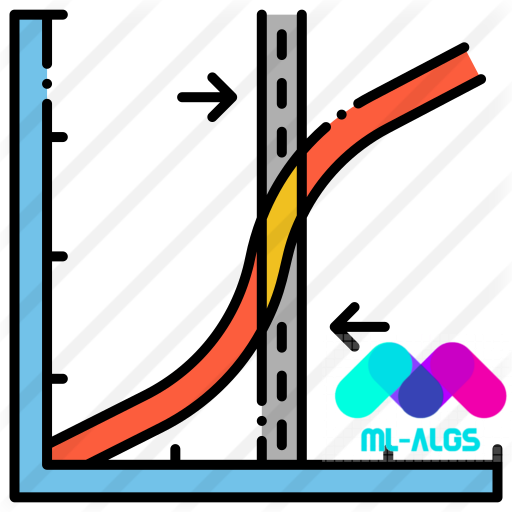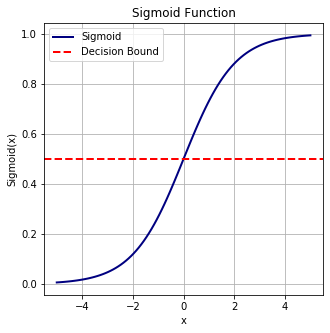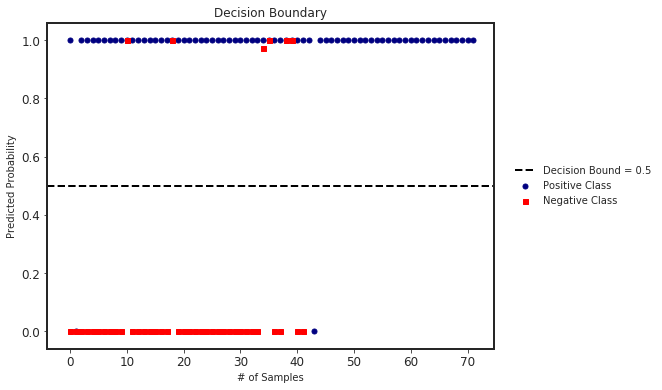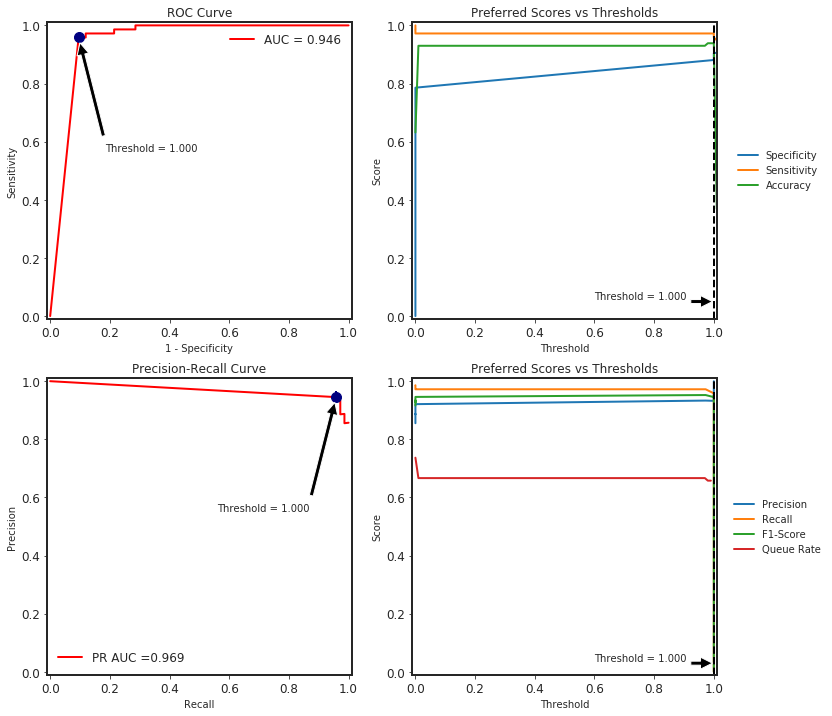# Introduction

### Sigmoid Function: $f(x) = \frac{1}{1 + exp(-x)}$

# loading libraries
import numpy as np
import matplotlib.pyplot as plt
import matplotlib as mpl
import seaborn as sns

from IPython.core.display import display, HTML
import warnings

sns.set_style("ticks")
mpl.rcParams["axes.linewidth"] = 2
mpl.rcParams["lines.linewidth"] = 2
warnings.filterwarnings("ignore")
display(HTML("<style>.container { width:95% !important; }</style>"))

def sigmoid(x):
"""
Returns sigmoid(x)
"""
return 1.0/(1 + np.exp(-x))

def plot_sigmoid(x):
"""
Plots sigmoid(x)
"""
fig, ax = plt.subplots(figsize=(5,5))
ax.plot(x, sigmoid(x), lw=2, c="navy", label="Sigmoid")
ax.axhline(0.5, lw=2, ls="--", c="red", label="Decision Bound")
ax.set(title="Sigmoid Function",
xlabel="x",
ylabel="Sigmoid(x)")
ax.grid(True)
ax.legend(loc=0)
plt.plot()

# define x to plot sigmoid(x)
x = np.linspace(-5, 5, 100)
plot_sigmoid(x)## The main algorithm to develop Logistic Regression is based on updating weights using Gradient Descent approach iteratively.

### - Update bias $b = b - lr \times db$ where $db = \frac{1}{N} \Sigma_{i=1}^{N}(\hat{y_i} - y_i)$

class LogisticRegression:
"""
Logistic Regression based on Gradient Descent
z = wx + b
y_hat = sigmoid(z) = sigmoid(wx + b) = 1/(1 + exp[-(wx+b)])
update rules: w = w - lr * dw
b = b - lr * db
where dw = 1/N sum(2x(y_hat - y))
db = 1/N sum(2(y_hat - y))
Parameters
----------
lr: float, optional (default=0.001)
Learning rate used of updating weights/bias
n_iters: int, optional (default=1000)
Maximum number of iterations to update weights/bias
weights: numpy.array, optional (default=None)
Weights array of shape (n_features, ) where
will be initialized as zeros
bias: float, optional (default=None)
Bias value where will be initialized as zero
"""

def __init__(self, learning_rate=0.001, n_iters=1000, weights=None, bias=None):
self.learning_rate = learning_rate
self.n_iters = n_iters
self.weights = None
self.bias = None

def fit(self, X_train, y_train):
"""
Train model using iterative gradient descent
Parameters
----------
X_train: numpy.array
Training feature matrix
y_train: 1D numpy.array or list
Training binary class labels [0, 1]
"""
# unpack the shape of X_train
n_samples, n_features = X_train.shape

# initialize weights and bias with zeros
self.weights = np.zeros(n_features)
self.bias = 0.0

# main loop
# self.loss = []
for _ in range(self.n_iters):
z = np.dot(X_train, self.weights) + self.bias
y_hat = self._sigmoid(z)

# update weights + bias
dw = (1.0 / n_samples) * 2 * np.dot(X_train.T, (y_hat - y_train))
db = (1.0 / n_samples) * 2 * np.sum(y_hat - y_train)

self.weights -= self.learning_rate * dw
self.bias -= self.learning_rate * db

return None

def predict_proba(self, X_test):
"""
Prediction probability of the test samples
Parameters
----------
X_test: numpy.array
Testing feature matrix
"""
z = np.dot(X_test, self.weights) + self.bias
y_pred_proba = self._sigmoid(z)

return y_pred_proba

def predict(self, X_test, threshold=0.5):
"""
Prediction class of the test samples
Parameters
----------
X_test: numpy.array
Testing feature matrix
threshold: float, optional (default=0.5)
Threshold to define predicted classes
"""
y_pred_proba = self.predict_proba(X_test)
y_pred = [1 if x >= threshold else 0 for x in y_pred_proba]

return y_pred

def plot_decision_boundary(self, X_test, y_test, threshold=0.5, figsize=(8, 6)):
"""
Plot the decision boundary
Parameters
----------
X_test: numpy.array
Testing feature matrix
y_test: 1D numpy.array or list
Testing targets
threshold: float, optional (default=0.5)
Threshold to define predicted classes
figsize: tuple, optional (default=(8,6))
Figure size
"""
# calc pred_proba
y_pred_proba = self.predict_proba(X_test)

# define positive and negative classes
pos_class = []
neg_class = []
for i in range(len(y_pred_proba)):
if y_test[i] == 1:
pos_class.append(y_pred_proba[i])
else:
neg_class.append(y_pred_proba[i])

# plotting
fig, ax = plt.subplots(figsize=figsize)
ax.scatter(
range(len(pos_class)),
pos_class,
s=25,
color="navy",
marker="o",
label="Positive Class",
)
ax.scatter(
range(len(neg_class)),
neg_class,
s=25,
color="red",
marker="s",
label="Negative Class",
)
ax.axhline(
threshold,
lw=2,
ls="--",
color="black",
label=f"Decision Bound = {threshold}",
)
ax.set_title("Decision Boundary")
ax.set_xlabel("# of Samples", fontsize=12)
ax.set_ylabel("Predicted Probability", fontsize=12)
ax.tick_params(axis="both", which="major", labelsize=12)
ax.legend(bbox_to_anchor=(1.2, 0.5), loc="center", ncol=1, framealpha=0.0)
plt.show()

def _sigmoid(self, z):
"""
Sigmoid Function
f(z) = 1/(1 + exp(-z))
"""

return 1.0 / (1 + np.exp(-z))


### This is part of the ML-Algs library which can be downloaded via PIP or from Git.

# you can simply do
!pip install mlalgs

# or cloning the project directly from GitHub
!git clone https://github.com/amirhessam88/ml-algs.git

# change directory to the project
%cd ml-algs

# installing requirements
!pip install -r requirements.txt

# loading libraries
from mlalgs.logistic_regression import LogisticRegression
from sklearn import datasets
from sklearn.model_selection import train_test_split
from sklearn.metrics import accuracy_score


### Sample breast cancer data for binary classification task. I have used stratifed train/test splits where test size is the 20\% of the data.

# loading data
data = datasets.load_breast_cancer()
X, y = data.data, data.target
X_train, X_test, y_train, y_test = train_test_split(X, y,
test_size=0.2,
shuffle=True,
random_state=1367,
stratify=y)


### Now, train the model using our algorithm.

# define model
clf = LogisticRegression()
# train model
clf.fit(X_train, y_train)
# predicted class
y_pred = clf.predict(X_test)
# predicted proba
y_pred_proba = clf.predict_proba(X_test)

# accuracy
accuracy_score(y_test, y_pred)

0.9298245614035088


### We can also plot the decision boundary and the predicted probabilities in each class.

clf.plot_decision_boundary(X_test, y_test)### We can also calculate all the binary classification metrics using SlickML library.

# simply install slickml via pip
!pip install slickml

# loading BinaryClassificationMetrics
from slickml.metrics import BinaryClassificationMetrics

# initialize the metric object
clf_metrics = BinaryClassificationMetrics(y_test, y_pred_proba)

Accuracy Balanced Accuracy ROC AUC PR AUC Precision Recall Average Precision F-1 Score F-2 Score F-0.50 Score Threat Score TP TN FP FN
Threshold = 0.500 | Average = Binary 0.930000 0.915000 0.946000 0.969000 0.921000 0.972000 0.943000 0.946000 0.962000 0.931000 0.897000 70 36 6 2
# plot roc, precision-recall with different thresholds calculations
clf_metrics.plot()